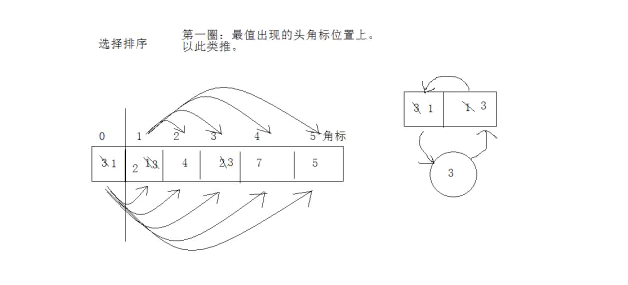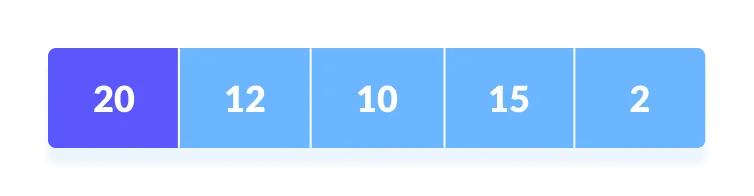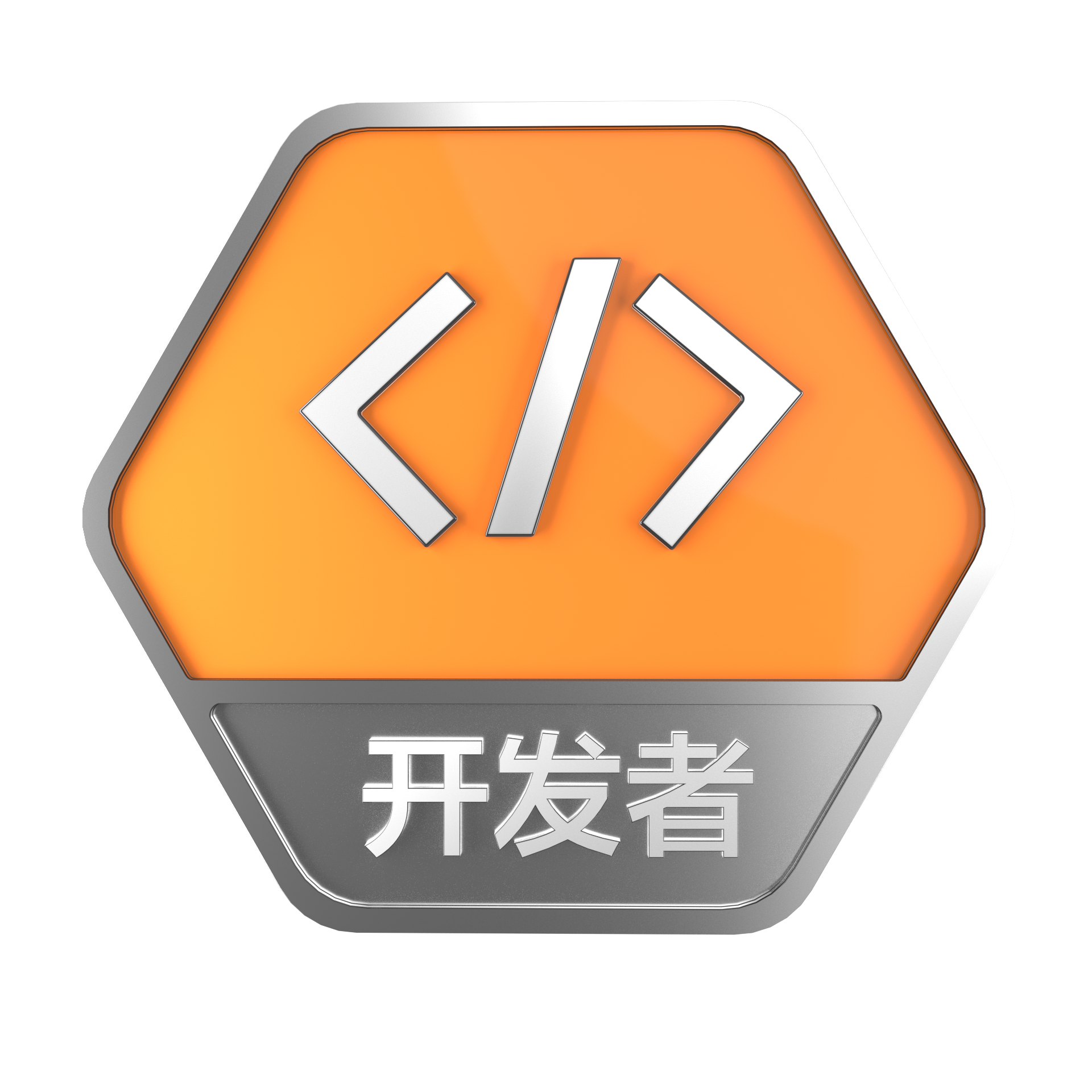# 从排序算法到TopK问题

+关注继续查看

## 二、冒泡

    private static float[] pickTopKByBubbleSort(float[] a, int k) {
int n = a.length;
for (int i = 0; i < k; i++) {
for (int j = 0; j < n - i - 1; j++) {
if (a[j] > a[j + 1]) {
float t = a[j];
a[j] = a[j + 1];
a[j + 1] = t;
}
}
}

float[] r = new float[k];
for (int i = 0; i < k; i++) {
r[i] = a[n - i - 1];
}
return r;
}

## 三、堆

     private static float[] pickTopKByHeapSort(float a[], int k) {
int n = a.length;
PriorityQueue<Float> queue = new PriorityQueue<>(n);
for (int i = 0; i < k; i++) {
queue.offer(a[i]);
}

for (int i = k; i < n; i++) {
if (a[i] > queue.peek()) {
queue.poll();
queue.offer(a[i]);
}
}

float[] r = new float[k];
for (int i = k - 1; i >= 0; i--) {
r[i] = queue.poll();
}
return r;
}

1、只放k个元素到堆中，然后遍历剩下的元素，和堆顶的元素（堆中最小的值）比较，
2、若大于堆顶，则取出堆顶的元素，插入当前元素；若小于等于堆顶，直接跳过；
3、最后依次取出堆中元素。

## 四、快排

    private static int partition(float[] a, int left, int right) {
int i = left;
int j = right;
float key = a[left];

while (i < j) {
while (i < j && a[j] <= key) {
j--;
}
a[i] = a[j];
while (i < j && a[i] >= key) {
i++;
}
a[j] = a[i];
}
a[i] = key;
return i;
}

private static void sort(float[] a, int left, int right) {
if (left >= right) {
return;
}
int index = partition(a, left, right);
sort(a, left, index - 1);
sort(a, index + 1, right);
}

1、通过一趟排序将要数据分割成独立的两部分，其中一部分的所有数据都比另外一部分的数据都要小；
2、然后再按此方法对这两部分数据分别进行第1步的操作（递归），以此达到整个数据变成有序序列。

    private static void sort(float[] a, int left, int right, int k) {
if (left >= right) {
return;
}
int index = partition(a, left, right);
int len = index - left + 1;
if (k < len) {
sort(a, left, index - 1, k);
} else if (k > len) {
sort(a, index + 1, right, k - len);
}
}

private static float[] pickTopKByQuickSort(float a[], int k) {
sort(a, 0, a.length - 1, k);
float[] r = new float[k];
for (int i = 0; i < k; i++) {
r[i] = a[i];
}
// 执行以上操作之后，r[]数组确实包含了top k的元素，但是不一定有序
// 如果仅仅是要求TopK而不需要有序，则不需要执行下面的排序函数
sort(r, 0, k - 1);
return r;
}

## 五、桶排序

    private static float[] pickTopKByBucketSort(float[] a, int k) {
int[] bucket = new int;

for (int i = 0; i < a.length; i++) {
int index = Math.round(a[i] * 100f);
bucket[index]++;
}

float[] r = new float[k];
int p = 0;
for (int i = bucket.length - 1; i >= 0 && p < k; i--) {
int c = bucket[i];
while (c > 0 && p < k) {
r[p++] = i / 100f;
c--;
}
}

return r;
}

## 六、测试

    public static void main(String[] args) {
int n = 100000;
int k = 100;
boolean success = true;

Random r = new Random();
for (int j = 0; j < 100; j++) {
float[] a = new float[n];
for (int i = 0; i < n; i++) {
float t = r.nextFloat() * 100f;
t = (int) (t * 100) / 100f;
a[i] = t;
}

float[] tmp = Arrays.copyOf(a, n);
float[] top1 = pickTopKByBubbleSort(tmp, k);

tmp = Arrays.copyOf(a, n);
float[] top2 = pickTopKByHeapSort(tmp, k);

tmp = Arrays.copyOf(a, n);
float[] top3 = pickTopKByQuickSort(tmp, k);

tmp = Arrays.copyOf(a, n);
float[] top4 = pickTopKByBucketSort(tmp, k);

if (!Arrays.equals(top1, top2)
|| !Arrays.equals(top1, top3)
|| !Arrays.equals(top1, top4)) {
success = false;
break;
}
}

if (success) {
System.out.println("success");
} else {
System.out.println("failed");
}
}

## 七、总结

• 冒泡的方法看起来时间复杂度是最高的，但是假如K很小，比如极端情况 , K=1，这时候冒泡的方法反而是最快的；
• 平均情况下，如果可以用的上桶排序（数据取值范围较小），那桶排序是最快的；
• 如果数值取值范围较小，而N很大（比方说100000），K又比较小（比方说100）时，运行发现堆的方法比快排的方法快。
原因猜测：范围小，数据多，应该有很多数值是重复的； 用堆的方法，堆会很快攒到Top的数值（即使还不是TopK），检索到那些小的数值的时候就不需要插入，从而检索后面的元素时，操作就只剩简单的遍历了。43 0【算法】选择排序
【算法】选择排序
13 041 01198 0882 0776 01346 0668 0995 0+关注

16

0